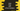# 4 different Java program to find the HCF or GCD of two numbers## Java program to find the HCF or GCD of two numbers:

Let’s learn how to find the HCF of two numbers in Java. HCF is also called GCD. The full form of HCF is Highest Common Factor and the full form of GCD is Greatest Common Divisor.

The HCF or GCD of two or more numbers is the largest non-zero common factor of these numbers. For example, the GCD of 8 and 12 is 4. Because

• The factors of 8 are 1, 2, 4, 8
• The factors of 12 are 1, 2, 3, 4, 6, 12

The highest common factor is 4.

Let me explain the algorithm before we start writing the program.

### Algorithm to find the HCF or GCD of two numbers:

The program will take the numbers as inputs from the user.

• Take the numbers as inputs from the user and assign the values to two different variables.
• Initialize one variable to hold the HCF value.
• Run one loop from 1 to the smaller value of the two numbers.
• Inside the loop, check if the current variable used in the loop can divide both numbers or not. If yes, assign this value to the HCF variable.
• Once the loop ends, the HCF variable will hold the required HCF or GCD.

Basically, we are using a loop from 1 to the smaller number and dividing both the numbers by the loop variable to find the GCD or HCF. The largest value that can divide both of these numbers is the GCD of the two numbers.

### Method 1: Java program to find the GCD or HCF by using a for loop:

The below Java program uses the above algorithm to find the GCD:

``````import java.util.Scanner;

public class Main {
public static void main(String[] args) {
int first, second, hcf = 1;
Scanner scanner = new Scanner(System.in);

System.out.println("Enter the first number: ");
first = scanner.nextInt();

System.out.println("Enter the second number: ");
second = scanner.nextInt();

for (int i = 2; i <= first || i <= second; i++) {
if (first % i == 0 && second % i == 0) {
hcf = i;
}
}

System.out.println("HCF: " + hcf);
}
}``````
• The integer variables first and second are used to hold the first and the second numbers, hcf is used to hold the calculated HCF. It is initialized as 1.
• This program uses a Scanner object to read the user input numbers.
• The for loop runs from i = 2. We have already defined hcf as 1. So, we need to find any value that can divide both the numbers and which is greater than 1.
• The value of hcf is updated if i can divide both the numbers.
• At the end of the program, it prints the calculated HCF value.

Sample result:

``````Enter the first number:
4
Enter the second number:
12
HCF: 4``````

### Method 2: Java program to find HCF or GCD by using a while loop:

We can easily convert the above program to use a while loop instead of a for loop. The only change we need is to initialize the variable i before the loop starts and increment its value at the end of each iteration of the loop.

``````import java.util.Scanner;

public class Main {
public static void main(String[] args) {
int first, second, hcf = 1, i = 2;
Scanner scanner = new Scanner(System.in);

System.out.println("Enter the first number: ");
first = scanner.nextInt();

System.out.println("Enter the second number: ");
second = scanner.nextInt();

while (i <= first || i <= second) {
if (first % i == 0 && second % i == 0) {
hcf = i;
}
i++;
}

System.out.println("HCF: " + hcf);
}
}``````

This program will print similar results.

### Method 3: Java program to find HCF with repeated subtraction:

This is another way to find HCF. We will run a while loop until the numbers become equal. On each iteration, it will subtract the smaller number from the bigger number and assign this to the bigger number variable.

``````import java.util.Scanner;

public class Main {
public static void main(String[] args) {
int first, second;
Scanner scanner = new Scanner(System.in);

System.out.println("Enter the first number: ");
first = scanner.nextInt();

System.out.println("Enter the second number: ");
second = scanner.nextInt();

while (first != second) {
if (first > second)
first = first - second;
else
second = second - first;
}

System.out.println("HCF: " + first);
}
}``````

The number variable holds the required HCF.

### Method 4: By using the Euclidean Algorithm:

This is one of the most efficient algorithms to find the GCD.

``````import java.util.Scanner;

public class Main {
static int findGCD(int first, int second){
if(second == 0)
return first;
return findGCD(second , first % second);
}

public static void main(String[] args) {
int first, second;
Scanner scanner = new Scanner(System.in);

System.out.println("Enter the first number: ");
first = scanner.nextInt();

System.out.println("Enter the second number: ");
second = scanner.nextInt();

System.out.println("GCD: " + findGCD(first, second));
}
}``````

This algorithm is based on a similar fact as the previous algorithm. If we keep subtracting the smaller number from the larger number, we will get the GCD once both become equal. We can also divide the first number by the second number and assign the remainder to the second number and assign the original second number to the first number. When the second number will become 0, the first number will hold the GCD value. This program will also give similar results.